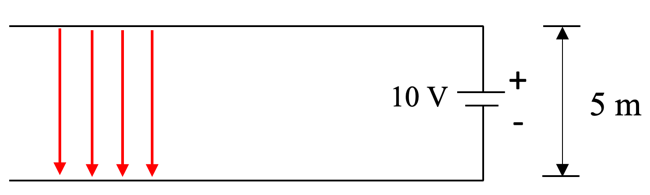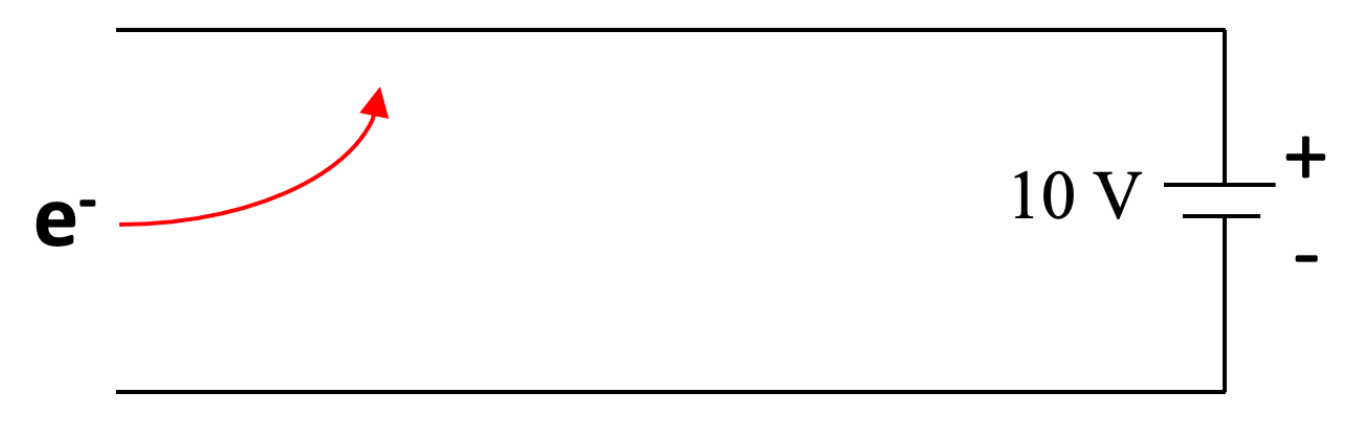# M6-S1: Charged Particle in an Electric Field

• ### investigate and quantitatively derive and analyse the interaction between charged particles and uniform electric fields, including: (ACSPH083)

• electric field between parallel charged plates E=V/d
• acceleration of charged particles by the electric field F_Net=ma, F=qE
• work done on the charge W=qV, W=qEd, K=1/2mv^2

### Electric Field Strength

• Two parallel charged plates connected to a potential difference produce a uniform electric field of strength:

E=V/d

where E is the electric field strength (Vm-1 or NC-1), V is the potential difference (voltage) in volts (V), and d is the perpendicular distance between the two parallel plates in metres (m).

• Note: ‘d’ is strictly the distance between two charged plates, it is not dependent on the position of a particle within the electric field.

• The direction of such an electric field always goes from the positively charged plate to the negatively charged plate (shown below).• Example: In the electric field above, the electric field strength is:

E=10/5=2 Vm-1 downwards

• A charged particle experiences a force when in an electric field.
• Positively charged particles are attracted to the negative plate
• Negatively charged particles are attracted to the positive plate
• The magnitude of this force is given by the equation:

F_E=qE

Where F is the force (N), q is the charge of the particle (C), and E is the electric field strength

• Direction of force depends on the nature of particle’s charge.
• The charge of the particle is either given by the question or provided in the reference sheet

• The electric field strength can therefore be also expressed in the form:

E=F/q

Since:

E=V/d

Therefore:

F/q=V/d

• By Newton’s second law (F=ma), any charged particle in an electric field experiences acceleration. Hence, their change in displacement increases with time (path of motion is curved not linear).### Work Done in an Electric Field

• Since the force acting on a charged particle can be determined by its charge (C), electric field strength (E), potential difference between charged plates (V) and distance between them (d), work done is expressed as such:

W=Fs

Substitute force with qE and displacement with distance between a pair of charged metal plates:

W=(qE)*(d)

W=qEd

Alternatively, can also be expressed in terms electric field strength and potential difference across the metal plates:

d=V/E

Thus,

W=qE*(V/E)

W=qV

where d is displacement and only equals to distance between two charged plates if the charged particle is moved from one plate to another. In other words, the second equation only applies when work is done to move a charged particle against the electric field. (diagram below)• Work done by electric field can be analysed by a change in kinetic energy of the charged particle

W=DeltaK = 1/2m(v-u)^2

Where u and v are initial and final velocities of the charged particle respectively.

Practice Question 1

An electron travelling at 50 m/s enters a uniform electric field created by a pair of parallel metal plates connected to a potential difference of 7 V.(a) Calculate the electric field strength. (1 mark)

(b) Calculate the acceleration experienced by the electron upon entering the electric field. (2 marks)

(c) After a short while, the electron is found stationary on the surface of the positive plate. Calculate the work done to move the electron from the positive plate to the negative plate. (1 mark)

### Qualitative Comparison

 Similarities Differences ·      Both experience a constant force and acceleration. Hence, their path of motion (parabolic) is similar ·      Motion of a charged particle in an electric field can also be resolved into vertical and horizontal components whereby the horizontal is constant. ·      Origin/cause of force/acceleration is difference ·      Charged particles also experience small and negligible amount of gravitational force ·      Direction of charged particle depends on nature of charge whereas direction of projectile motion is always downwards (towards centre of Earth) ·      Magnitude of force/acceleration is governed by different parameters

### Quantitative Comparison

• Charged particles experience very little and negligible amount of gravitational force.
• For example, for an electron on the surface of Earth it experiences gravitational force of magnitude:
F_g=(GMm)/d^2

F_g=((6.67xx10^-11)(6.0xx10^24)(9.109xx10^-31))/(6371xx10^3)^2

F=9.0xx10^-30 N towards the centre of Earth

• Compared with typical electric fields, the contribution from electric force is much more significant than gravitational force.

﻿Next section: ﻿Charged Particles in Magnetic Fields